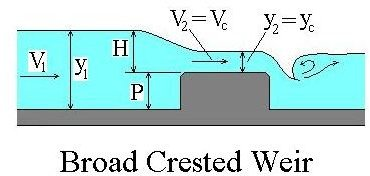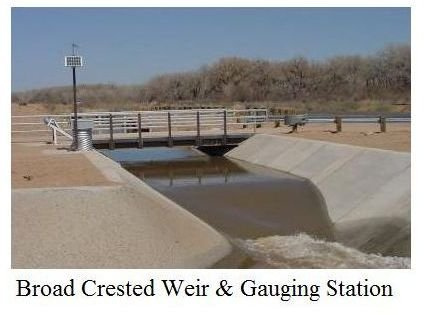# Critical Flow Broad Crested Weir: Calculating Flow Rate of Critical Flow Over the Weir Crest

Broad crested weirs are widely used for flow measurement and regulation of water depth in rivers, canals and other natural open channels. Broad crested weir flow rate calculations can be made with a rather simple equation if the weir height is great enough to cause critical flow over the weir crest.

For a broad crested weir with less than critical velocity over the weir crest, the discharge coefficient will vary from 2.3 to 3.3 depending upon the breadth of the weir crest and the head over the weir.

If there is critical flow, then the equation to use is Q = 1.6 L H3/2, where Q is the open channel flow rate in cfs, L is the weir length (channel width) in ft, and H is the head over the weir in ft, as shown in the diagram below.

## General Broad Crested Weir ConfigurationA broad crested weir is normally a flat topped obstruction which extends across the entire channel, as shown in the picture at the right. Alongitudinal section of a typical broad crested weir is shown in the diagram at the left. The head over the weir, H, the height of the weir, P, the approach velocity and depth, V1 and y1, and the velocity and depth on the weir crest, V2 and y2, are all shown in the diagram. A key feature of a properly operating broad crested weir is critical flow over the weir crest. This is shown in the diagram by noting that V2 = Vc and y2 = yc. The next section discusses determination of the minimum weir height to ensure that critical flow will occur over the weir crest.

Image credit: Middle Rio Grande Conservancy District

## Minimum Height Requirement for a Critical Flow Broad Crested Weir

The water velocity will increase whenever the flow in an open channel passes over an obstruction like a broad crested weir, because of the decrease in cross-sectional flow area. Within limits, the higher the obstruction, the greater the water velocity will be going over the obstruction. If the approach flow is subcritical, then the flow over a broad crested weir will become critical at some particular weir height. That height needed to give critical flow over the weir crest can be calculated using some basic hydraulics equations, Using the terminology in the broad crested weir figure above, along with B for the width of the channel, the energy equation becomes:

y1 + V12/2g = y2 + P + V22/2g

From the definition of average velocity in an open channel, assuming that the channel is approximately rectangular:

V1 = Q/y1B and Vc = Q/ycB

From the fact that the specific energy is a minimum for critical flow conditions:

yc = [Q2/gB2]1/3

If the channel width, B, the flow rate through the channel, Q, and the approach depth, y1, are known, the depth required to give critical flow over the broad crested weir crest can be calculated with the above three equations. If a broad crested weir has the minimum height needed for critical flow, then the simple equation, Q = 1.6 L H3/2, can be used for flow rate calculations over that broad crested weir.

This is illustrated with example calculations in the next section. In order to ensure critical flow over the weir for all flow conditions, the maximum anticipated flow rate through the channel should be used to calculate the required weir height, P.

## Example Minimum Height Calculation

Problem Statement: Consider an open channel, 20 ft wide, with a maximum anticipated flow rate of 350 cfs at a flow depth of 5 ft. What is the minimum height needed for a broad crested weir to ensure critical flow over the weir crest?

Solution: Using the equations from the previous section, with Q = 350 cfs, y1 = 5 ft, and B = 20 ft:

V1 = Q/y1B = 350/(5)(20) = 3.5 ft/sec

yc = [Q2/gB2]1/3 = [3502/(32.2)(202)]1/3 = 2.12 ft

Vc = Q/ycB = 350/(2.12)(20) = 8.25 ft/sec

P = y1 + V12/2g – y2 – V22/2g = 5 + 0.19 – 2.12 – 1.06 = 2.01 ft

If the broad crested weir height is at least 2.01 ft, then the equation, Q = 1.6 L H3/2, can be used to calculate the flow rate (up to 350 cfs), from a measured value for the head over the weir, H.

For Excel spreadsheets that can be downloaded to make broad crested weir flow rate calculations in either U.S. or S.I. units, see the article, “Excel Spreadsheets for Broad Crested Weir Calculations.”

Read more from this author on related topics by visting his blog: Engineering Excel Spreadsheets

## References

References for Further Information:

1. U.S. Dept. of the Interior, Bureau of Reclamation, Water Measurement Manual, 2001 revised, 1997 third edition.

2. Munson, B. R., Young, D. F., & Okiishi, T. H., Fundamentals of Fluid Mechanics, 4th Ed., New York: John Wiley and Sons, Inc, 2002.

3. Chanson, Hubert, Hydraulics of Open Channel Flow: An Introduction – Basic principles, Sediment Motion, Hydraulic Modeling, Design of Hydraulic Structures, Second Edition, New York, Elsevier, 2004.

## This post is part of the series: Open Channel Flow Measurement

Open channel flow rate measurement is usually done by measuring a change in water depth. It can be done with a weir or flume. Common types are the sharp crested weir (including V-notch weir, rectangular weir, and cipolletti weir), the broad crested weir, the Parshall flume and venturi flume.